Скачать презентацию Option Pricing basic principles Value boundaries Simple arbitrage

• Количество слайдов: 12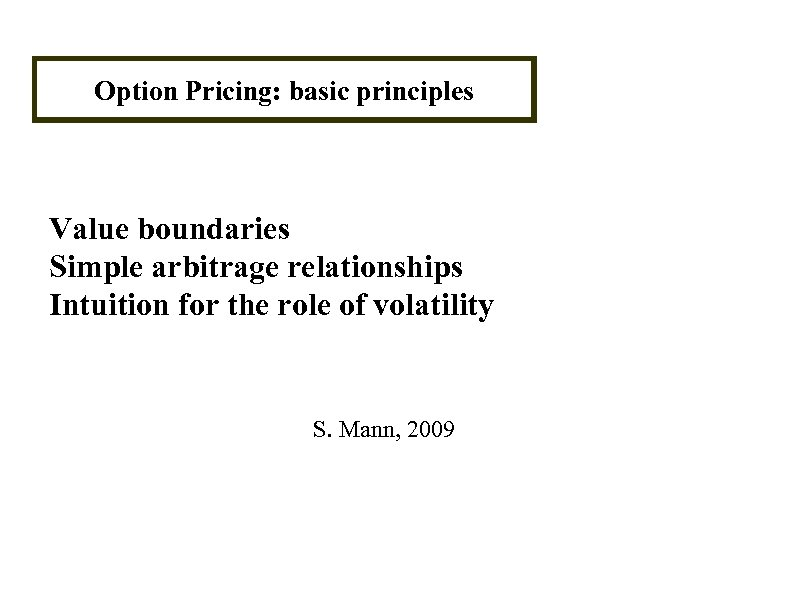Option Pricing: basic principles Value boundaries Simple arbitrage relationships Intuition for the role of volatility S. Mann, 2009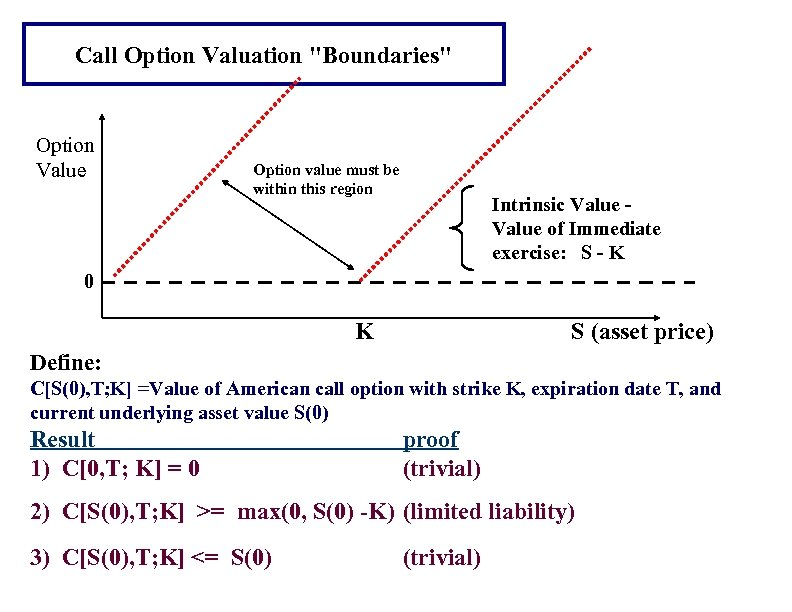Call Option Valuation "Boundaries" Option Value Option value must be within this region Intrinsic Value of Immediate exercise: S - K 0 K S (asset price) Define: C[S(0), T; K] =Value of American call option with strike K, expiration date T, and current underlying asset value S(0) Result 1) C[0, T; K] = 0 proof (trivial) 2) C[S(0), T; K] >= max(0, S(0) -K) (limited liability) 3) C[S(0), T; K] <= S(0) (trivial)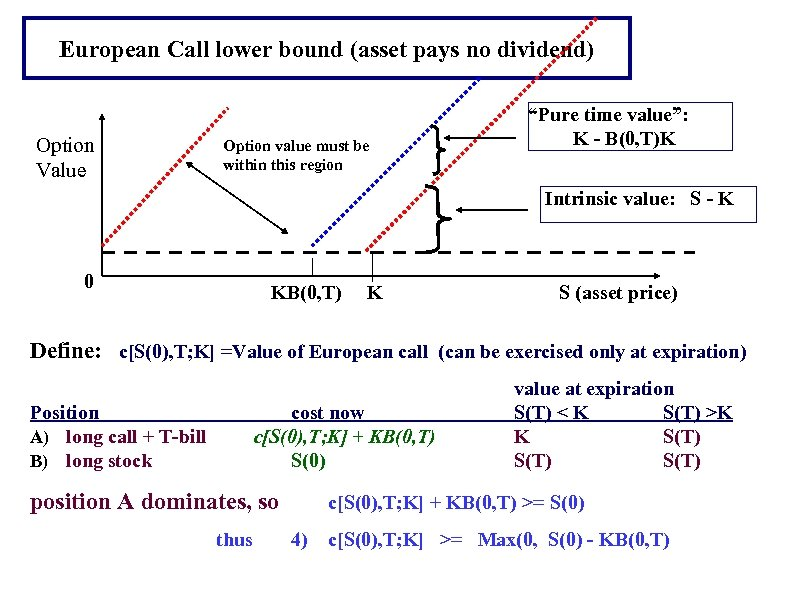European Call lower bound (asset pays no dividend) Option Value Option value must be within this region “Pure time value”: K - B(0, T)K Intrinsic value: S - K 0 KB(0, T) K S (asset price) Define: c[S(0), T; K] =Value of European call (can be exercised only at expiration) Position A) long call + T-bill B) long stock cost now c[S(0), T; K] + KB(0, T) S(0) position A dominates, so thus value at expiration S(T) < K S(T) >K K S(T) c[S(0), T; K] + KB(0, T) >= S(0) 4) c[S(0), T; K] >= Max(0, S(0) - KB(0, T)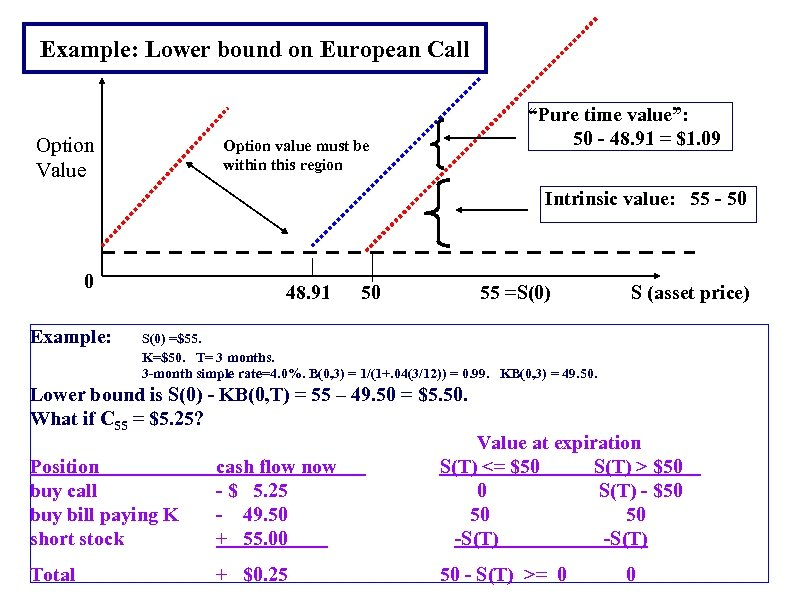Example: Lower bound on European Call Option Value “Pure time value”: 50 - 48. 91 = \$1. 09 Option value must be within this region Intrinsic value: 55 - 50 0 Example: 48. 91 50 55 =S(0) S (asset price) S(0) =\$55. K=\$50. T= 3 months. 3 -month simple rate=4. 0%. B(0, 3) = 1/(1+. 04(3/12)) = 0. 99. KB(0, 3) = 49. 50. Lower bound is S(0) - KB(0, T) = 55 – 49. 50 = \$5. 50. What if C 55 = \$5. 25? Position buy call buy bill paying K short stock cash flow now - \$ 5. 25 - 49. 50 + 55. 00 Value at expiration S(T) <= \$50 S(T) > \$50 0 S(T) - \$50 50 50 -S(T) Total + \$0. 25 50 - S(T) >= 0 0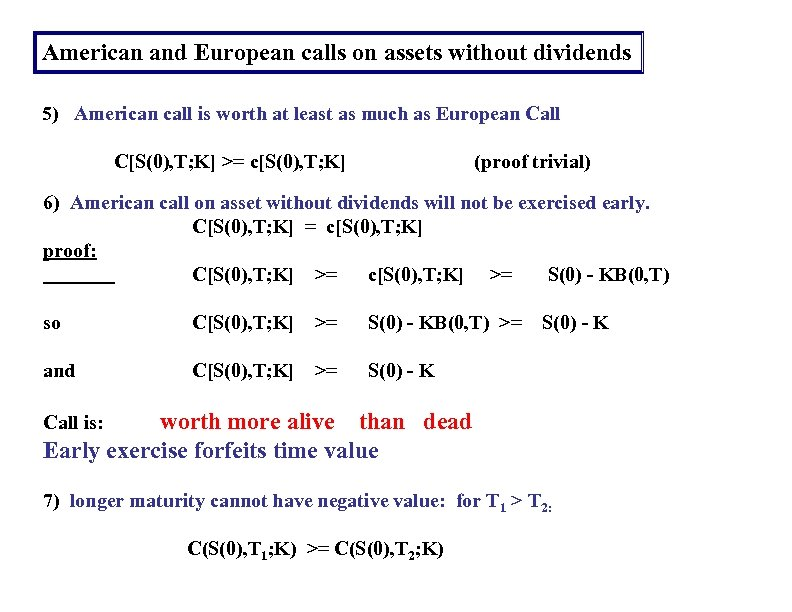American and European calls on assets without dividends 5) American call is worth at least as much as European Call C[S(0), T; K] >= c[S(0), T; K] (proof trivial) 6) American call on asset without dividends will not be exercised early. C[S(0), T; K] = c[S(0), T; K] proof: C[S(0), T; K] >= c[S(0), T; K] >= S(0) - KB(0, T) so C[S(0), T; K] >= S(0) - KB(0, T) >= S(0) - K and C[S(0), T; K] >= S(0) - K worth more alive than dead Early exercise forfeits time value Call is: 7) longer maturity cannot have negative value: for T 1 > T 2: C(S(0), T 1; K) >= C(S(0), T 2; K)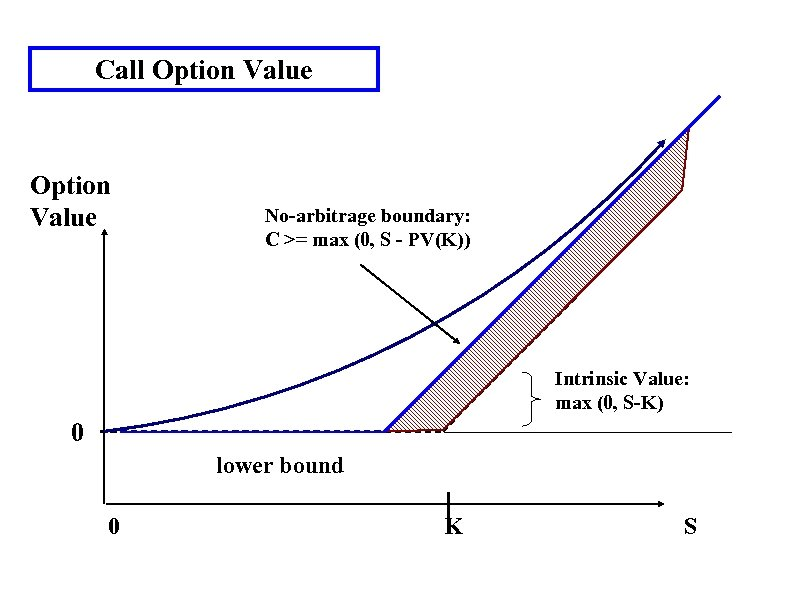Call Option Value No-arbitrage boundary: C >= max (0, S - PV(K)) Intrinsic Value: max (0, S-K) 0 lower bound 0 K S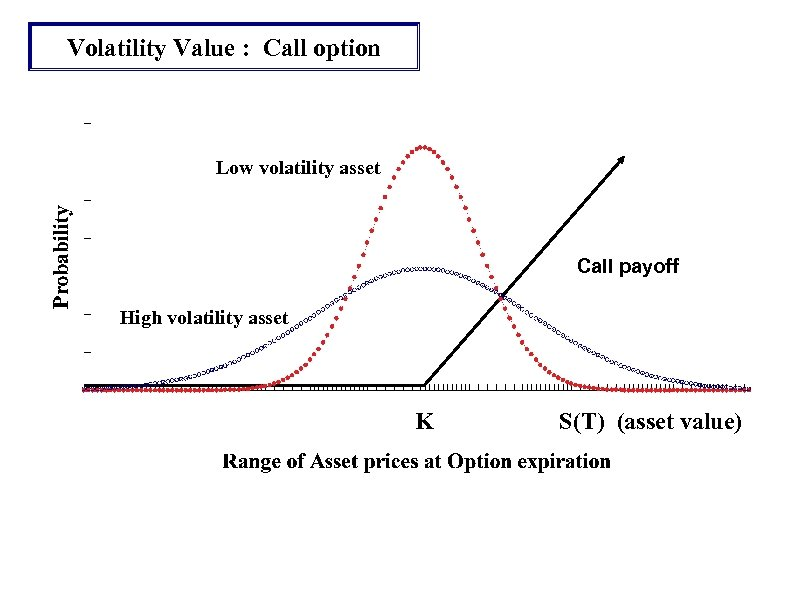Volatility Value : Call option Low volatility asset Call payoff High volatility asset K S(T) (asset value)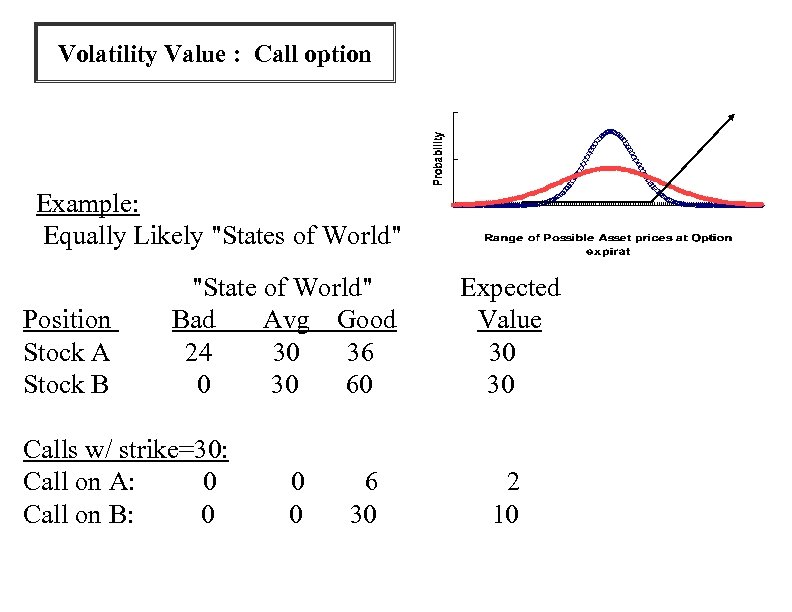Volatility Value : Call option Example: Equally Likely "States of World" Position Stock A Stock B "State of World" Bad Avg Good 24 30 36 0 30 60 Calls w/ strike=30: Call on A: 0 Call on B: 0 0 0 6 30 Expected Value 30 30 2 10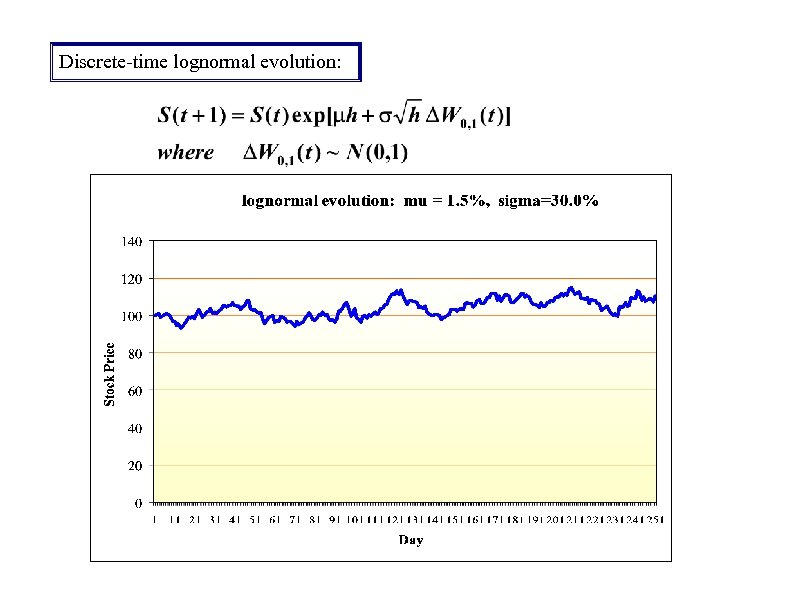Discrete-time lognormal evolution: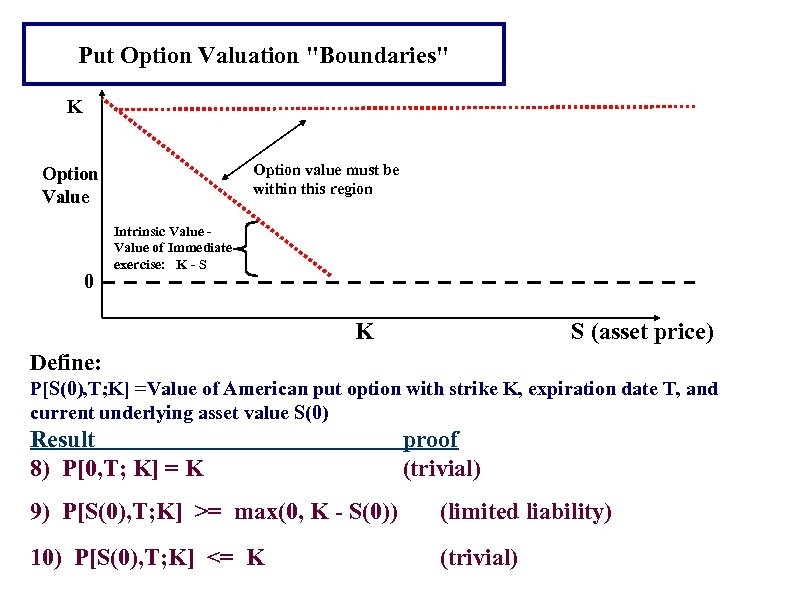Put Option Valuation "Boundaries" K Option value must be within this region Option Value 0 Intrinsic Value of Immediate exercise: K - S K S (asset price) Define: P[S(0), T; K] =Value of American put option with strike K, expiration date T, and current underlying asset value S(0) Result 8) P[0, T; K] = K proof (trivial) 9) P[S(0), T; K] >= max(0, K - S(0)) (limited liability) 10) P[S(0), T; K] <= K (trivial)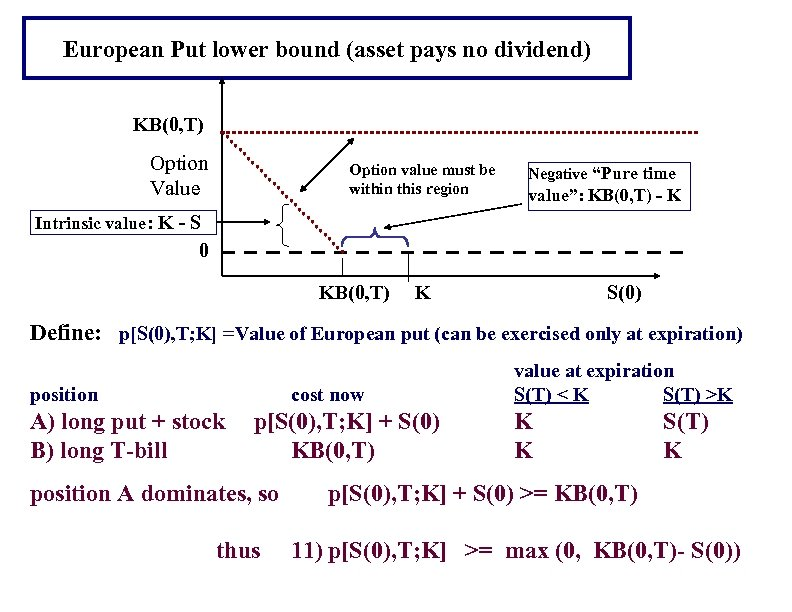European Put lower bound (asset pays no dividend) KB(0, T) Option Value Option value must be within this region Negative “Pure time value”: KB(0, T) - K Intrinsic value: K - S 0 KB(0, T) K S(0) Define: p[S(0), T; K] =Value of European put (can be exercised only at expiration) position cost now A) long put + stock B) long T-bill p[S(0), T; K] + S(0) KB(0, T) position A dominates, so thus value at expiration S(T) < K S(T) >K K K S(T) K p[S(0), T; K] + S(0) >= KB(0, T) 11) p[S(0), T; K] >= max (0, KB(0, T)- S(0))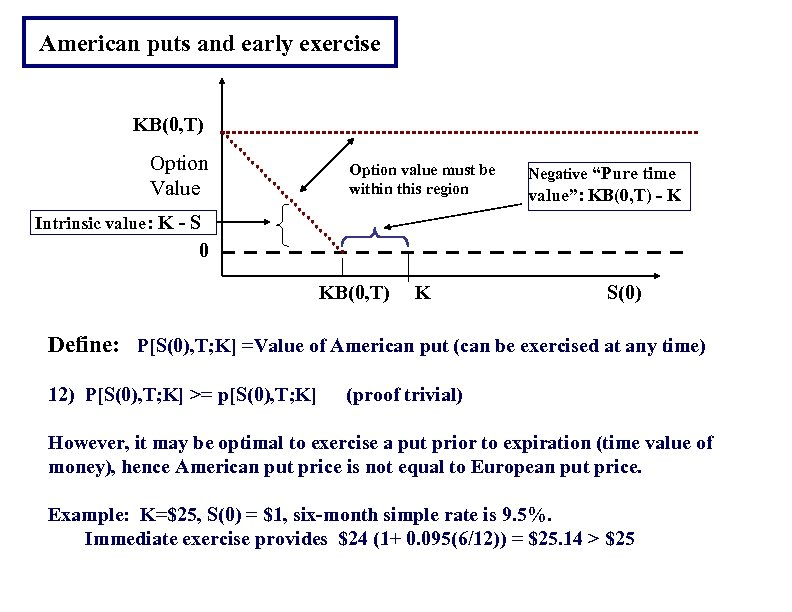American puts and early exercise KB(0, T) Option Value Option value must be within this region Negative “Pure time value”: KB(0, T) - K Intrinsic value: K - S 0 KB(0, T) K S(0) Define: P[S(0), T; K] =Value of American put (can be exercised at any time) 12) P[S(0), T; K] >= p[S(0), T; K] (proof trivial) However, it may be optimal to exercise a put prior to expiration (time value of money), hence American put price is not equal to European put price. Example: K=\$25, S(0) = \$1, six-month simple rate is 9. 5%. Immediate exercise provides \$24 (1+ 0. 095(6/12)) = \$25. 14 > \$25